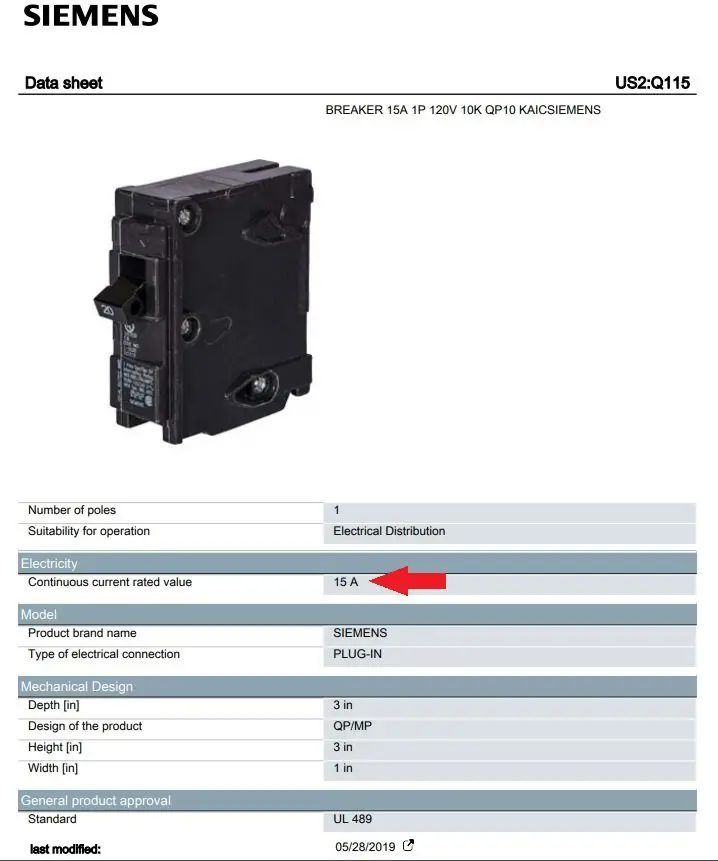# What are the Max Watts on a 15/20 Amp Circuit?

What are the max watts on a 15 amp circuit or 20 amp circuit before the breaker will trip?

Let’s do the math.

Watts = Volts * Amps

Most homes in the United States have outlet voltages around 120V.

So on a 15 amp circuit, the maximum watts are 15A*120V =  1800 watts.

## 80% Rated Circuit Breakers

Many 15A breakers nowadays can handle 1800 watts (100% load) continuously like this one from Siemens:However, some circuit breakers are rated at 80% of their current rating for continuous loads.

The NEC (National Electric Code) defines a continuous load as “a load where the maximum current is expected to continue for three hours or more.”

Section 384-16(c) of the NEC also states that a standard OCPD (overcurrent protection device) can be loaded to only 80% of its rating for continuous loads.

In this case, the max watts on a 15 amp circuit with a standard breaker are 0.8*1800 = 1440 watts.

## 20 Amp Circuit Breaker

The math is similar for a 20 amp circuit breaker.

Here the maximum watts are 20A*120V =  2400 watts for a 100% continuous current rated circuit breaker.

On a standard 20A circuit breaker rated for 80%, the maximum watts are 0.8*20A*120V = 1920 watts.